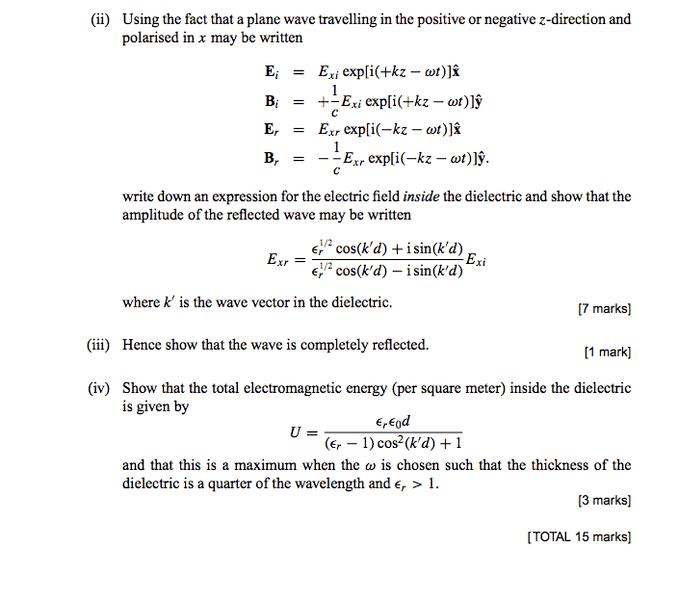# Plane Wave at Barrier

• bananabandana

## Homework Statement

Sorry for the dull question. Problem is as shown/attached## Homework Equations

The waves in part ii) are traveling in a HIL dielectric of permittivity ##\epsilon_{r}## from ##0 <z<d## and then hit an ideal metal boundary at ##z=d##.

## The Attempt at a Solution

I figure this should be quite obvious! The total field in the dielectric ##\vec{E_{D}}## is the superposition of the reflected and incident waves. At ##z=d## the field parallel to the boundary must be 0 -ideal metal is ideal conductor and so electrons can move to precisely counteract the field. We also know (from applying Faraday's law to a small loop around the boundary) that the parallel electric field must be continuous across the boundary. i.e

$$\vec{E_{d}} =E_{D}\hat{\mathbf{x}}= \bigg(E_{xi}exp[i(kz-\omega t)] + E_{xr} exp[i(-kz-\omega t) ]\bigg)\hat{\mathbf{x}}$$
[n.b - the frequency of the incident and reflected waves has to be the same, else we couldn't satisfy the boundary condition for all t,] So:
$$E_{D}(z=d) =0 \implies E_{xi} \big[cos(kd) +isin(kd)\big] =-E_{xr} \big[cos(kd)-isin(kd)\big]$$
$$E_{xr} = -\frac{ cos(kd)+isin(kd)}{cos(kd)-isin(kd)} E_{xi}$$
Which is obviously not the result I'm meant to get! What has gone wrong?

It looks to me that the given formula for the plane wave is for free space for z<0. So, you have the first get the expression for the wave inside the dielectric. That is where you will get the ##\epsilon_r## and ##k'## terms.